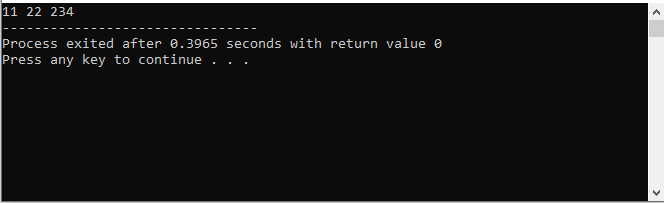×

Search anything:

# Extract integers from string and store in vector using C++

#### C++ Software EngineeringIndian Technical Authorship Contest starts on 1st July 2023. Stay tuned.

Reading time: 10 minutes | Coding time: 5 minutes

### Introduction

In this article we will learn how to extract integers from a string and store it in a vector using C++.

First let's define vectors and strings. Vectors are more robust versions of arrays and less error-prone compared to arrays. Unlike arrays where it's static and their size is needed to be declared beforehand, vectors are dynamic and can resize itself. The benefits of using vectors is it can allocate space for growth, however, because of this they also consume more memory compared to using arrays. Strings are one-dimensional arrays that represent sequences of characters like integers and letters.

Now let's look into a sample problem:

``````   Input: String = "There are 5 dogs and 3 cats."
Output: 5 3

Input: String = " OpenGenus 11 22 234 test"
Output: 11 22 234
``````

To extract the integers from the string we will be using stringstream. Stringstream is a stream class in C++ that let you operate on strings. Stringstream lets you read, extract, or insert into a string object. We will pass the string to a stringstream and using a loop we can look into each word and check if it's an integer. If an integer is detected it will be appended to a vector. The loop will continue until it reached the end of the string.

### Algorithm

1. Pass the string to stringstream
2. In a loop, go through each word
3. Check if the word is an integer or not
4. Store values in the vector

### Complexity

• Worst case time complexity: `Θ(N)`
• Average case time complexity: `Θ(N)`
• Best case time complexity: `Θ(1)`

### Implementations

``````
#include <iostream>
#include <sstream>
#include <vector>
using namespace std;

int main()
{
stringstream ss;
string str = " OpenGenus 11 22 234 test";

// store the string to string stream
ss << str;

//declare vector
vector< int > intValues;

string temp;
int number;
while (!ss.eof()) {

//for getting each word in the string
ss >> temp;

// check for integers
if (stringstream(temp) >> number){
// if it is an integer value will be pushed to the vector
intValues.push_back(number);
}

}
// print values inside the vector
for (int i=0; i<intValues.size(); i++){
cout<<intValues[i] << " ";
}
return 0;
}

``````

Output:### Applications

• Can be used to get integer values from string input from users.
• Vectors can be used when the size of values to be stored is unknown.

### Question 1

What command do you use to append values into a vector.
front()
push_back()
pop_back()
assign()
push_back() function is used to push elements into a vector from the back.

### Question 2

Are vectors static or dynamic?
Static
Depends on implementations
Both
Dynamic
Vectors are dynamics and can resize itself.

With this article at OpenGenus, you must have the complete idea of how to Extract integers from string and store in vector using C++.